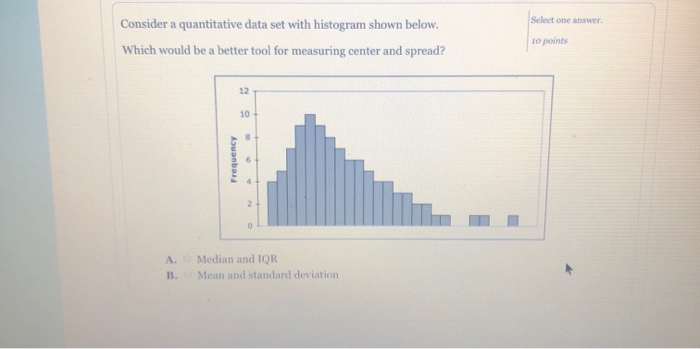# Question Solved1 AnswerSelect one answer Consider a quantitative data set with histogram shown below. Which would be a better tool for measuring center and spread? 10 points 12 10 Frequency A. Median and IQR B. Mean and standard deviationJ4MSQ4 The Asker · Probability and StatisticsTranscribed Image Text: Select one answer Consider a quantitative data set with histogram shown below. Which would be a better tool for measuring center and spread? 10 points 12 10 Frequency A. Median and IQR B. Mean and standard deviation
More
Transcribed Image Text: Select one answer Consider a quantitative data set with histogram shown below. Which would be a better tool for measuring center and spread? 10 points 12 10 Frequency A. Median and IQR B. Mean and standard deviation